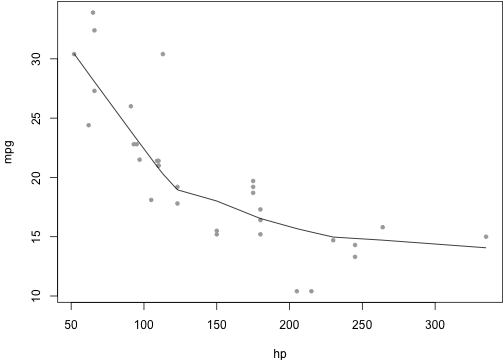# A Minimal Example for Markdown This is a minimal example of using **knitr** to produce an _HTML_ page from _Markdown_. ## R code chunks {r setup} # set global chunk options: images will be 7x5 inches opts_chunk$set(fig.width=7, fig.height=5)  Now we write some code chunks in this markdown file: {r computing} x <- 1+1 # a simple calculator set.seed(123) rnorm(5) # boring random numbers  We can also produce plots: {r graphics} par(mar = c(4, 4, .1, .1)) with(mtcars, { plot(mpg~hp, pch=20, col='darkgray') lines(lowess(hp, mpg)) })  ## Inline code Inline R code is also supported, e.g. the value of x is r x, and 2 × π = r 2*pi. ## Math LaTeX math as usual:$f(\alpha, \beta) \propto x^{\alpha-1}(1-x)^{\beta-1}$. ## Misc You can indent code chunks so they can nest within other environments such as lists. 1. the area of a circle with radius x {r foo} pi * x^2  2. OK, that is great To compile me, use {r compile, eval=FALSE} library(knitr) knit('knitr-minimal.Rmd')  ## Conclusion Markdown is super easy to write. Go to **knitr** [homepage](http://yihui.name/knitr) for details. # A Minimal Example for Markdown This is a minimal example of using knitr to produce an HTML page from Markdown. ## R code chunks # set global chunk options: images will be 7x5 inches opts_chunk$set(fig.width = 7, fig.height = 5)


Now we write some code chunks in this markdown file:

x <- 1 + 1  # a simple calculator
set.seed(123)
rnorm(5)  # boring random numbers

##  -0.56048 -0.23018  1.55871  0.07051  0.12929


We can also produce plots:

  par(mar = c(4, 4, 0.1, 0.1))
with(mtcars, {
plot(mpg ~ hp, pch = 20, col = "darkgray")
lines(lowess(hp, mpg))
})## Inline code

Inline R code is also supported, e.g. the value of x is 2, and 2 × π = 6.2832.

## Math

LaTeX math as usual: $f(\alpha, \beta) \propto x^{\alpha-1}(1-x)^{\beta-1}$.

## Misc

You can indent code chunks so they can nest within other environments such as lists.

1. the area of a circle with radius x
pi * x^2

##  12.57

1. OK, that is great

To compile me, use

library(knitr)
knit("knitr-minimal.Rmd")


## Conclusion

Markdown is super easy to write. Go to knitr homepage for details.